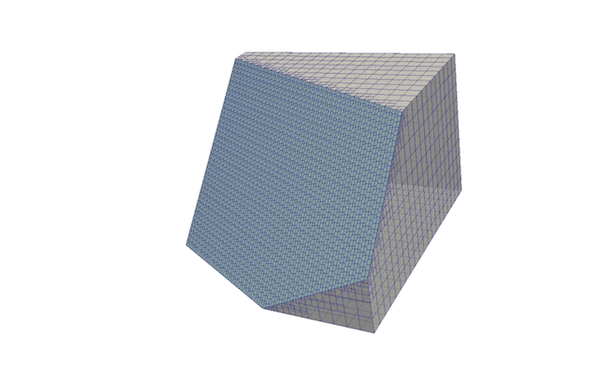An infinite plane. The plane can be used to cut-out part of the domain, where the mesh in the plane normal direction will be preserved. The plane can be specified according to:

• pointAndNormal Plane is defined by point and normal vector

• embeddedPoints Plane is defined by 3 points

• planeEquation Employs the algebraic plane equation:

$ax + by + cz + d = 0$

## Usage🔗

Plane defined by point and a normal vector:

plane
{
type            searchablePlane;
planeType       pointAndNormal;

pointAndNormalDict
{
basePoint       (1 1 1);
normal          (0 1 0);
}
}


Plane defined by 3 points on the plane:

plane
{
type            searchablePlane;
planeType       embeddedPoints;

embeddedPointsDict
{
point1          (1 1 1);
point2          (0 1 0);
point3          (0 0 1)
}
}


Plane defined by plane equation:

plane
{
type            searchablePlane;
planeType       planeEquation;

planeEquationDict
{
a  0;
b  0;
c  1; // to create plane with normal towards +z direction ...
d  2; // ... at coordinate: z = 2
}
}